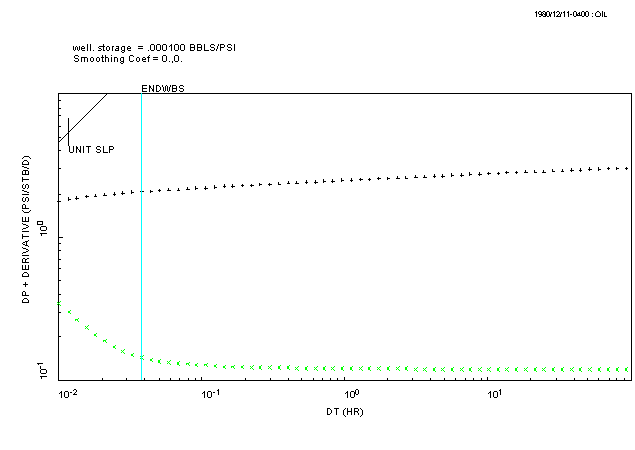## ESTIMATE THE END OF WELL-BORE STORAGE EFFECTS

Select the ANALYSIS pull-down menu in PIE and select the "DIAGNOSTIC" option. This will display a set of graphics functions (the floating 'toolbar' at the bottom of the main window) that allow you to draw lines on the plot.

It is not possible to make a reliable estimate of the storage coefficient when the data does not show a unit-slope or the well-bore storage coefficient is changing. In this case, the time at which well-bore storage effects end should be estimated by entering a calculated storage coefficient. Follow these steps to enter the C(min) value we calculated earlier:

1. Select the button for the UNITSL function.
2. Pick any point to the left of the data on the derivative plot
3. Turn off the UNITSL function by clicking on the button again. (Note how the button pushes in and out).
4. Use the mouse to click on the "well. Storage" value shown on the plot. Note the prompt to enter a new value at the top of the plot: enter the value of C(min) calculated previously.

The end of well-bore storage effects is calculated from the C(min) value and a vertical line is drawn to show this time on the plot. The end result of these steps should look something like this:WRITE DOWN the number of log-cycles on the time axis between the "ENDWBS" line and the end of the test data (the above figure shows a value of just under 3.5 log-cycles). We need this value later.

Q: Do all the data points lie to the RIGHT of the unit-slope line?